Disclaimer: This is an example of a student written essay.

Any scientific information contained within this essay should not be treated as fact, this content is to be used for educational purposes only and may contain factual inaccuracies or be out of date.

# Literature Review on Offshore Wind Power and Impacts of Waves

 ✅ Paper Type: Free Essay ✅ Subject: Environmental Sciences ✅ Wordcount: 2854 words ✅ Published: 3rd Nov 2020

Literature Review

Offshore Wind Power

Offshore wind power is the generation and supply of electric power through the harvest of wind energy at offshore locations such as lakes, oceans and sheltered coastal areas. According to Renewables Global Status Report 2019 (REN21, 2019), wind power has second most installed capacity among all renewable energies, contributing remarkably to low carbon energy production. Over the years, the ratio of offshore wind energy to onshore wind energy is increasing in terms of installed capacity, with 1% before 2010 and 4% in 2018 (Global Wind Energy Council, 2019). Further understanding of wave impact on offshore foundations are required to ensure that improvement of the substructure is in pace with the increasing trend of the offshore wind turbine size. According to(Röckmann, Lagerveld and Stavenuiter, 2017), the maintenance cost of offshore wind farms is 38%, which highest among all cost. Therefore, a more efficient offshore wind structure design would be needed to further reduce this cost. With that in mind, this research will focus on wave impacts, specifically wave forces, pressure and runups on offshore substructures.

Offshore Foundations

Offshore foundations can be categorized into fixed foundation or floating foundation. Among all the installed offshore wind turbines, the widely used foundation is the monopile foundation (74.5%) and the jacket foundation (24.5%) (Wind Europe, 2019). Although various other foundations have been exploited these two remain to be favored by the industry. Hence, in this research, the focus will be on monopile foundation. Monopiles with a 6 meters diameter are often used for locations with water depth of 0-30m, with some going beyond that range.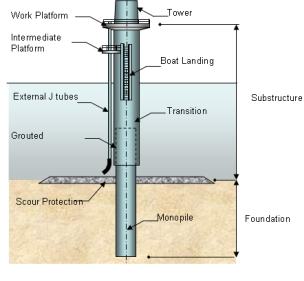Figure 1: Monopile foundation for offshore wind turbines. Image from (Wind Energy The Facts, n.d.)

Linear Wave Theory

Airy (Linear) wave theory is the fundamental theory to understand surface gravity waves. It describes surface gravity waves motion as sinusoidal using the velocity potential approach and linearized boundary conditions. The basic assumption for linear wave theory is the fluid being inviscid and irrotational. From the linear wave theory, for monochromatic waves:

Regular wave surface profile,

η(x,t)=Acos⁡(kx-ωt)

Velocity potential, Φ= Hg2ωcosh⁡k(d+z)cosh⁡kdsin⁡(kx-ωt)

Instantaneous horizontal velocity, u= πHT[cosh⁡k(d+z)sinh⁡kd]cos⁡(kx-ωt)

Instantaneous vertical velocity, w= πHT[sinh⁡k(d+z)sinh⁡kd]sin⁡(kx-ωt)

Where  x  =  Horizontal position of water particle

t = Time

k = 2πL (Wave number)

ω = 2πT (Wave angular frequency)

H = Vertical distance from crest to trough

g = Gravitational acceleration

d = Water depth

z = Vertical distance of water particle from still water level

These equations give us the required perimeter to calculate the regular wave forces on a monopile foundations.

Stokes Wave Theory (Non-linear wave theory)

However, to look into the violent wave impacts on the monopile foundations of offshore wind turbines, we would have to consider non-linear wave conditions. Due to the fact the foundations are exposed to real random ocean waves which are affected by factors such as vorticity and turbulence, the linear wave theory is not sufficient to explain the wave interaction between ocean waves and the foundation. Stokes (Stokes, 1847) used the approach of perturbation expansion to obtain the 2nd order stokes wave. Since then, higher order of stokes wave have been developed such as the fifth order wave theory by (Lars and James, 1960). This allows us to obtain the surface wave profile for waves with higher order harmonics, which can be used to calculate the non-linear wave induced force on the structure. Other than the high-order stokes wave theory, (Kristiansen and Faltinsen, 2017) used the FNV theory(Faltinsen, Newman and Vinje, 1995) to calculate the horizontal wave forces for higher order waves. In this method, discrepancies due to flow separation is found.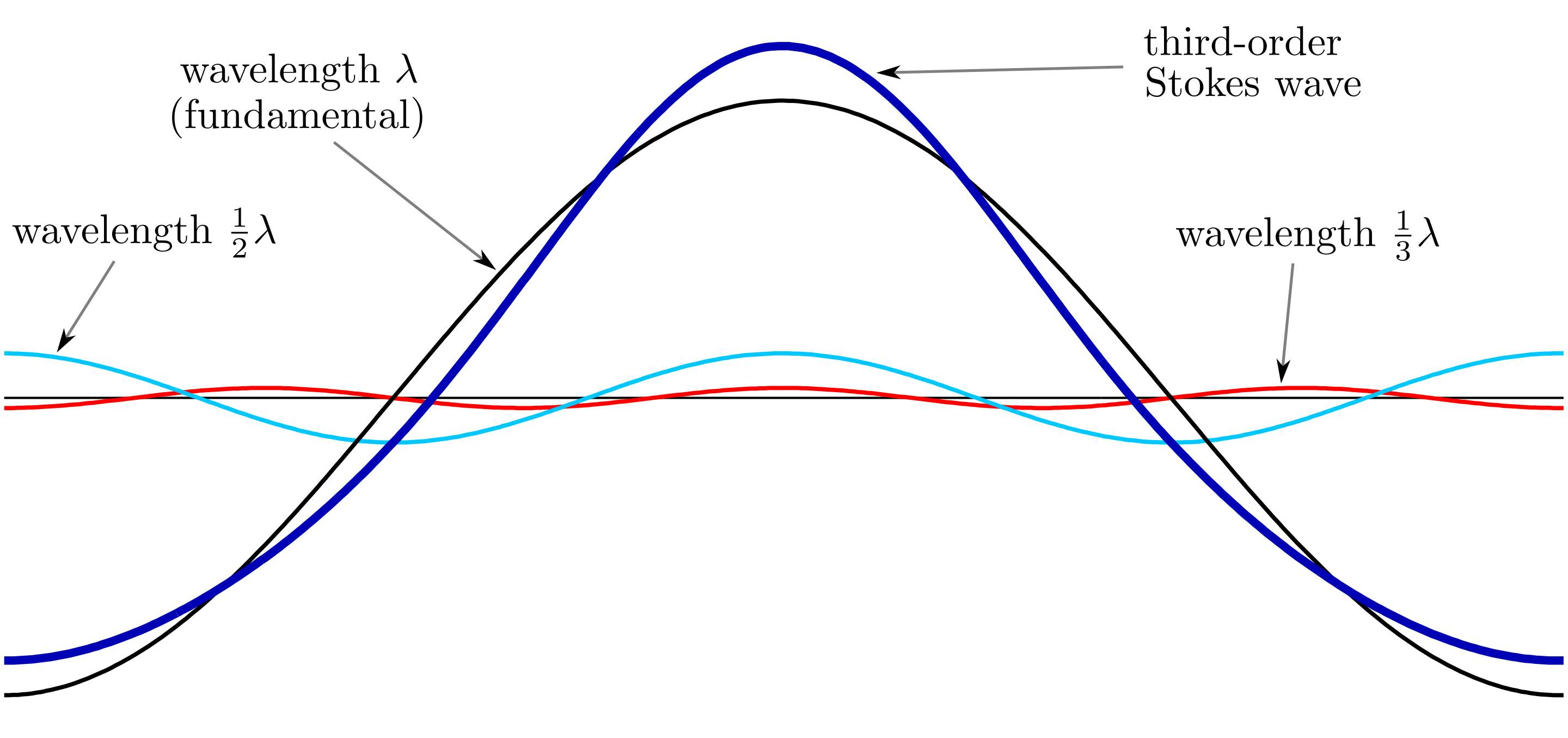Figure 2: Third order stokes wave surface profile. [Image from Wikipedia]

High-order non-linear waves should be of concern when designing offshore wind turbine foundations as it can contribute to as high as 60% of the total wave loading on the foundation structure(Chen et al., 2018).

Wave forces on monopile foundations

In general, the Morison Equation(Morison, Johnson and Schaaf, 1950) is the fundamental equation used to calculate the theoretical wave forces acting on a monopile foundation for non-breaking waves. The Morison Equation shows the force components acting on a submerged cylinder which the diameter of the cylinder not disrupting the wave significantly (i.e. causing wave reflection and scattering). The Morison Equation is valid for small bodies with Dλ<0.2, where D is the diameter and  λ is the wavelength.

The Morison Equation for inline forces parallel to wave direction:

F= Cd2ρDu2+ CmρπD24dudt

Where   Cd  = Drag coefficient

ρ = Fluid density

D = Diameter of the monopile

u = Instantaneous horizontal velocity of water particle

Cm = Coefficient of mass

The first term, FD in the Morison Equation is the drag force caused by the formation of wake and boundary layer separation as the waves interacts with the cylinder. The wave particle motion behaves as a continuous unsteady flow. The flow velocity and acceleration exert a force on the body of the cylinder. Cd is the drag coefficient which is dependent on the body’s shape and surface roughness, flow orientation and Reynolds number, Re. Through dimensional analysis, it is found that Cd is a function of Re. However, the form of the function is not able to be determined from dimensional analysis. Hence, the relationship between Cd and Re is determined through experimental results, which is empirical.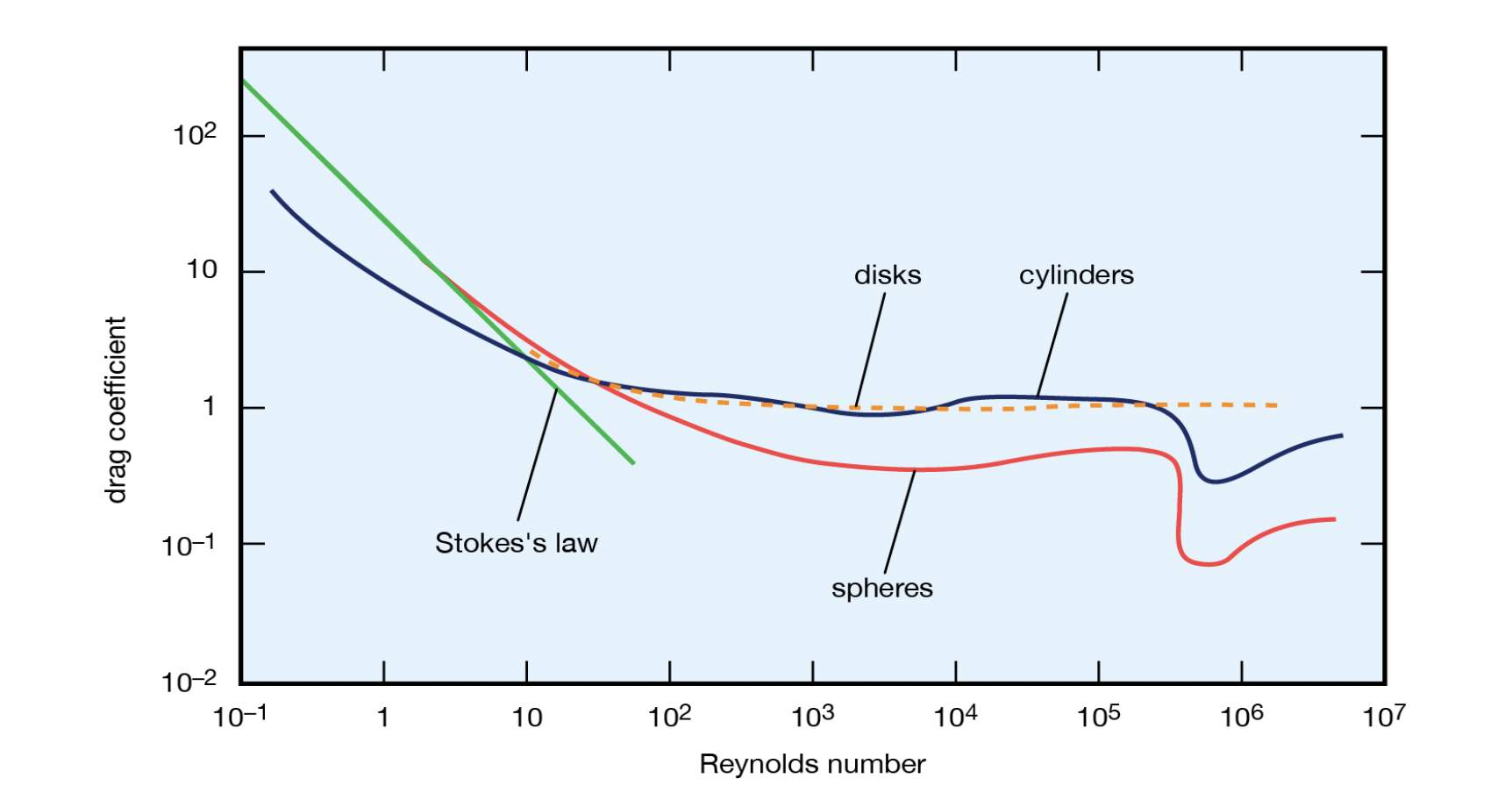Figure 3: Graph of Cd against Re for various shapes. Image from (Encyclopædia Britannica, 2019)

The second term, FM in the Morison Equation is a result of inertia force on the body. It is a function of the fluid density, acceleration, body shape and volume. It has two components, which is the Froude-Krylov force and the added-mass force. When the unsteady wave flow accelerates past the body, the pressure gradient around the body that allows the acceleration to happen induces a net force on the body. This is known as the Froude-Krylov force. The added-mass component is a result of the body causing local disturbance to the wave flow. The mass coefficient, Cm=1+ Ca, where  Ca is the added-mass coefficient, is a function of fluid density, body shape, acceleration and volume. The values Ca are also derived from experimental data and is dependent on flow orientations and body shapes. For circular cylinder with a flow normal to the axis, the value of Ca is 1.0. Ca values for other body shapes and flow orientation can be found from (Sarpkaya, Isaacson and Wehausen, 1982).

The total wave forces acting on a vertical cylindrical body, FTcan be obtained by integrating the Morison Equation:

FT= ∫-d0F dz

Where  d = height of cylindrical body from seabed

The Morison Equation provides a fundamental understanding of wave forces acting on submerged bodies, which subsequently leads to understanding of other behaviors such as wave pressures on submerged bodies. However, the Morison Equation still has its limitations. Since Reynolds Number is a function of velocity, it varies as the flow accelerates past the body as due to the changing velocity. The change in flow velocity also varies the drag coefficient relatively. With both velocity and drag coefficient varying in the accelerating flow, the drag force term in the Morison Equation can fluctuate greatly (Sorensen, 2006). If this is considered in the calculations, a very complicated drag force pattern can be expected on the body. Other than that, the two coefficients used in the equation, Cd and Cm are both determined empirically but not analytically. The value of the coefficients might not be suitable for some applied cases if the boundary conditions are different than that used in the experiments (i.e. the equation is not applicable to every flow regime). For the Morison Equation to be used, the assumed flow field past the body has to be reasonable (i.e. the body is small in relations to the wave particle orbit dimension). Also, the Morison Equation does not account for effects of breaking waves.

Other than using analytical method, numerical model to investigate the interaction between wave and structures are found to be accurate for waves of 4th order (Chen et al., 2014).

Breaking Waves

Breaking waves describe waves which its increase in amplitude or energy gain reaches a critical level, becomes unstable and dissipates the vast amount of wave energy into turbulence. When this happens, the wave energy of the system is clustered near the wave crest and spatial spread of energy occurs(Faltinsen, 1990). Occurrence of breaking waves are dependent on the site water depth, seabed slope, wave height, wave period and wave steepness. Breaking waves can be categorized into 4 types: Spilling, Plunging, Surging, and Collapsing.

Our academic experts are ready and waiting to assist with any writing project you may have. From simple essay plans, through to full dissertations, you can guarantee we have a service perfectly matched to your needs.

Plunging breaker waves occurs during a sudden or steep change in ocean floor depth. The wave crest becomes much steeper, sometimes to vertical, curls and fall over the trough. During this process, air might be trapped and compressed within the drop of the breaking wave, hence increasing the impact force. Plungers causes violent impact on offshore wind turbine foundations as the focused wave energy are dissipated over a small area of the substructure, with the duration in the order of milliseconds(Faltinsen, 1990). This results in slamming force and pressure to be exerted on the foundations of offshore wind turbines. Plunging breaker waves are of interest as it is likely to occur with the wind conditions at offshore location and it has the most violent impact on structures.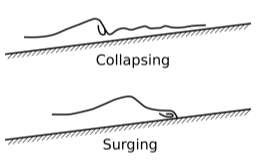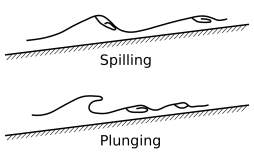Types of breaking waves. [Image from wikipedia]

When the wave breaks, the model used to describe wave behaviors becomes invalid. Hence, the Morison equation used to calculate the wave force has to be modified in order to account for the slamming force from the plunging breaker waves(Chella, Tørum and Myrhaug, 2012). An extra term that accounts for the slamming force from the breaking waves:

Modified Morison Equation, FTotal= FD+FM+FS

Slamming Force, FS=0.5ρCsDCb2ληb

Where  Cs = Slamming force factor

Cb = Breaking wave celerity

λ = Curling factor ≈ 0.5 (Wienke, Sparboom and Oumeraci, 2004)

ηb = Height of wave runup on structure from still water level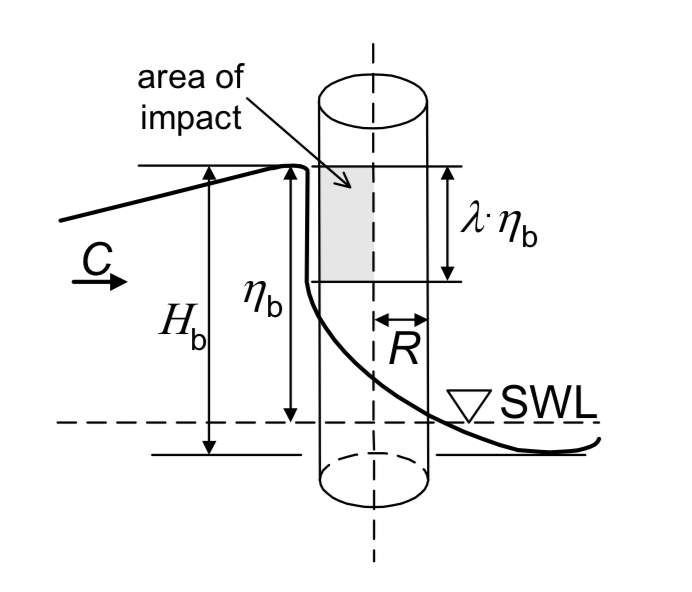Schematic diagram of the slamming force on slender cylinder. [Image from (Wienke, Sparboom and Oumeraci, 2004)]

Summary

The offshore wind industry is showing some interesting trend over the years: Significant reduction in levelized cost of electricity, increasing wind turbine size and capacity and increasing size of windfarms. The increasing trend of share of offshore to onshore wind is expected to continue for the future. With the efficiency of offshore wind turbines directly associated with its size, it is clear that stronger foundations would be needed. Although there are different kinds of foundations being experimented, monopiles are still the most widely favored foundation type. Therefore, this research will focus on monopile foundations of offshore wind turbines.

The airy wave theory is the core understanding of regular wave behavior however it is not sufficient for practical cases. The offshore wind turbines are exposed to random sea waves which can be better predicted using the non-linear wave theory, which accounts for the subharmonic effects of the waves. It is found out that theoretical wave loadings are generally calculated using both the Morison Equation and the wave theory. The Morison Equation accounts for the drag and inertial components of the wave but another slamming component has to be added into account for the slamming effects should breaking waves are considered.

Other than regular and non-regular waves, breaking waves are also of interest as it exerts a large localized force over a short amount of time on the structure upon breaking. This produces a huge impact force on the structure.

The intended outcome of this research is to understand the interaction between waves, local bed morphology and offshore structures (monopile foundations). Thereby hopefully to be able to apply the results and knowledge to the design of offshore monopile foundations to increase its efficiency and performance, hence reducing the cost.

Experiments are carried out to investigate the interaction between a cylindrical body and various wave condition. Analysis of experimental data and theoretical values will be compared and investigated to provide further understanding.

References

• Chella, M.A., Tørum, A. and Myrhaug, D., 2012. An Overview of Wave Impact Forces on Offshore Wind Turbine Substructures. Energy Procedia, 20, pp. 217-226.
• Chen, L.F., Zang, J., Hillis, A.J., Morgan, G.C.J. and Plummer, A.R., 2014. Numerical investigation of wave-structure interaction using OpenFOAM. Chen , L F , Zang , J , Hillis , A J , Morgan , G C J & Plummer , A R 2014 , ' Numerical investigation of wave-structure interaction using OpenFOAM ' , Ocean Engineering , vol. 88 , pp. 91-109 . https://doi.org/10.1016/j.oceaneng.2014.06.003 ISSN:0029-8018.
• Chen, L.F., Zang, J., Taylor, P.H., Sun, L., Morgan, G.C.J., Grice, J., Orszaghova, J. and Tello Ruiz, M., 2018. An experimental decomposition of nonlinear forces on a surface-piercing column: Stokes-type expansions of the force harmonics. Journal of Fluid Mechanics, 848, pp. 42-77.
• Faltinsen, O.M., 1990. Sea loads on ships and offshore structures. Cambridge: Cambridge : Cambridge University Press.
• Faltinsen, O.M., Newman, J.N. and Vinje, T., 1995. Nonlinear wave loads on a slender vertical cylinder. Journal of Fluid Mechanics, 289, pp. 179-198.
• Kristiansen, T. and Faltinsen, O.M., 2017. Higher harmonic wave loads on a vertical cylinder in finite water depth.
• Lars, S. and James, H., 1960. FIFTH ORDER GRAVITY WAVE THEORY. Coastal Engineering Proceedings, 1(7).
• Morison, J.R., Johnson, J.W. and Schaaf, S.A., 1950. The Force Exerted by Surface Waves on Piles. Journal of Petroleum Technology, 2(05), pp. 149-154.
• Röckmann, C., Lagerveld, S. and Stavenuiter, J., 2017. Operation and Maintenance Costs of Offshore Wind Farms and Potential Multi-use Platforms in the Dutch North Sea. Aquaculture Perspective of Multi-Use Sites in the Open Ocean: The Untapped Potential for Marine Resources in the Anthropocene. Cham: Springer International Publishing, pp. 97-113.
• Sarpkaya, T., Isaacson, M. and Wehausen, J.V., 1982. Mechanics of Wave Forces on Offshore Structures. Journal of Applied Mechanics, 49(2), p. 466.
• Sorensen, R.M., 2006. Basic Coastal Engineering. Third Edition ed. Boston, MA: Boston, MA: Springer US.
• Wienke, J., Sparboom, U.W.E. and Oumeraci, H., 2004. THEORETICAL FORMULAE FOR WAVE SLAMMING LOADS ON SLENDER CIRCULAR CYLINDERS AND APPLICATION FOR SUPPORT STRUCTURES OF WIND TURBINES.

View all

## DMCA / Removal Request

If you are the original writer of this essay and no longer wish to have your work published on UKEssays.com then please:

Related Services

Prices from

£124

Approximate costs for:

• 1000 words
• 7 day deliveryHumanity University

Dedicated to your worth and value as a human being!

Related Lectures